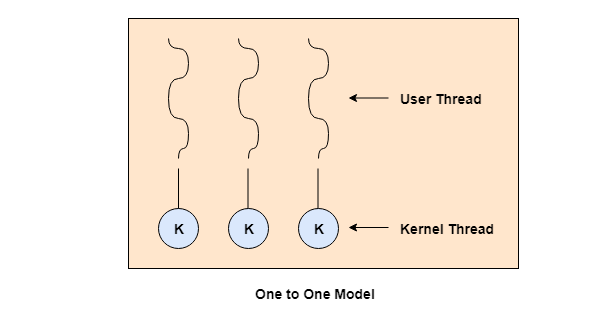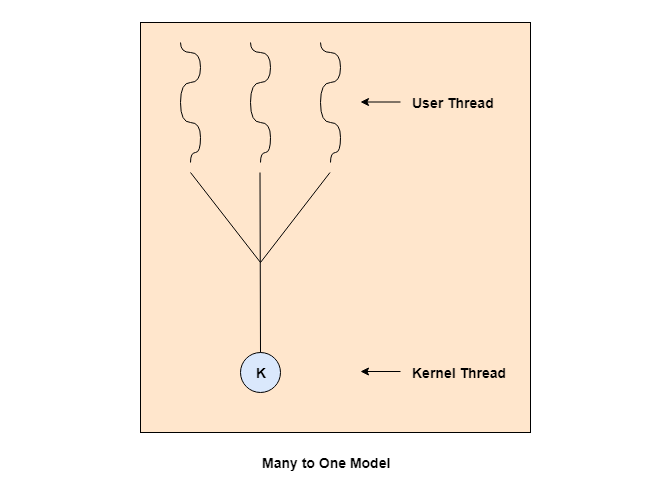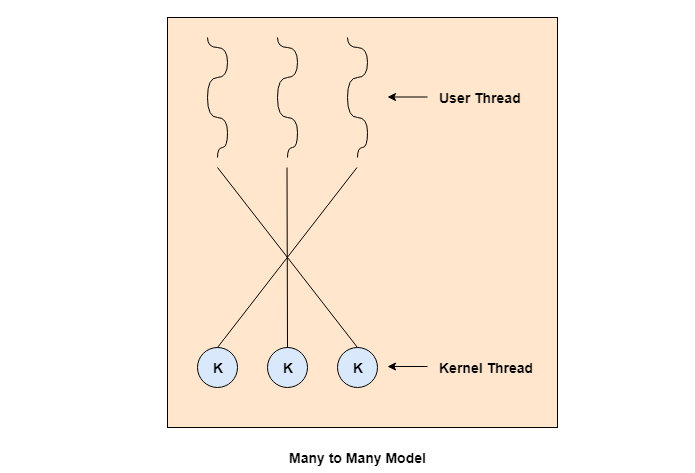Multithreading allows the execution of multiple parts of a program at the same time. These parts are known as threads and are lightweight processes available within the process. Therefore, multithreading leads to maximum utilization of the CPU by multitasking.

The main models for multithreading are one to one model, many to one model and many to many model. Details about these are given as follows −

## One to One Model

The one to one model maps each of the user threads to a kernel thread. This means that many threads can run in parallel on multiprocessors and other threads can run when one thread makes a blocking system call.

A disadvantage of the one to one model is that the creation of a user thread requires a corresponding kernel thread. Since a lot of kernel threads burden the system, there is restriction on the number of threads in the system.

A diagram that demonstrates the one to one model is given as follows −## Many to One Model

The many to one model maps many of the user threads to a single kernel thread. This model is quite efficient as the user space manages the thread management.

A disadvantage of the many to one model is that a thread blocking system call blocks the entire process. Also, multiple threads cannot run in parallel as only one thread can access the kernel at a time.

A diagram that demonstrates the many to one model is given as follows −## Many to Many Model

The many to many model maps many of the user threads to a equal number or lesser kernel threads. The number of kernel threads depends on the application or machine.

The many to many does not have the disadvantages of the one to one model or the many to one model. There can be as many user threads as required and their corresponding kernel threads can run in parallel on a multiprocessor.

A diagram that demonstrates the many to many model is given as follows −